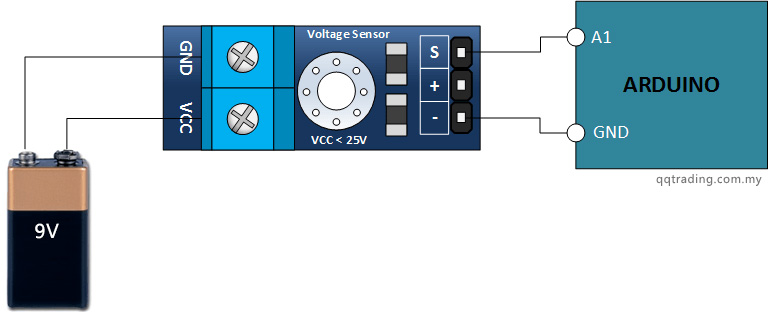# Voltage Sensor Module (Up to 25V)

• Was  RM10.50
• ## RM5.00

• Product Code: Voltage Sensor Module
• Availability: In Stock

A simple but very useful module which uses a potential divider to reduce any input voltage by a factor of 5. This allows you to use the analogue input of a microcontroller to monitor voltages much higher than it capable of sensing.

For example with a 0V - 5V analogue input range, you are able to measure a voltage up to 25V.

This module also includes convenient screw terminals for easy and secure connection of a wire.

This module is based on a principle of resistive voltage divider design, can make the red terminal connector input voltage to 5 times smaller. Arduino analog input voltages up to 5v, the voltage detection module input voltage not greater than 5Vx5=25V (if using a 3.3V systems, input voltage not greater than 3.3Vx5=16.5V).

Arduino AVR chips have 10-bit AD, so this module simulates a resolution of 0.00489V (5V/1023), so the minimum voltage of input voltage detection module is 0.00489Vx5=0.02445V.

Specification:

• Divider ratio: 5:1
• Resistor Tolerance: 1%
• Max input voltage: 25V
• Resistor Value: 30K/7.5K Ohm

Pinout:

INPUT:

• GND –  This is where you connect the low side of the voltage you are measuring.   * Caution:  This is the same electrical point as your Arduino ground.
• VCC –  The is where you connect the high side of  the voltage you are measuring (0 - 25V)

OUTPUT:

• – (or minus):  This connects to your Arduino ground.
• +:  This is not connected.  It does absolutely nothing.

## The Connections

Find yourself a 9 volt battery and connect it,  your voltage sensor module and Arduino as shown below.## The Sketch

Enter the following sketch, upload it and go to town.   If you open your Arduino serial monitor you will be able to see the voltage.

```/*
DC Voltmeter Using a Voltage Divider
Based on Code Created By
T.K.Hareendran
*/

float vout = 0.0;
float vin = 0.0;
float R1 = 30000.0; //
float R2 = 7500.0; //
int value = 0;
void setup(){
Serial.begin(9600);
Serial.print("DC VOLTMETER");
}
void loop(){
vout = (value * 5.0) / 1024.0; // see text
vin = vout / (R2/(R1+R2));

Serial.print("INPUT V= ");
Serial.println(vin,2);
delay(500);
}

```

## Write a review

Note: HTML is not translated!